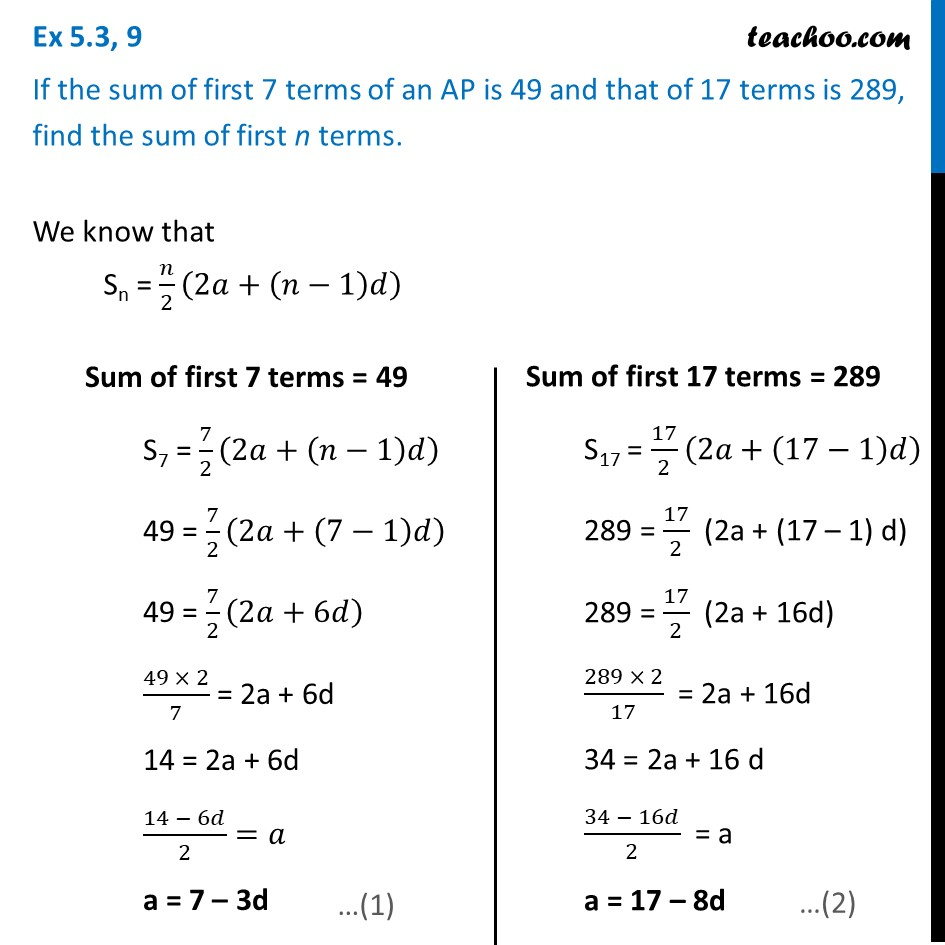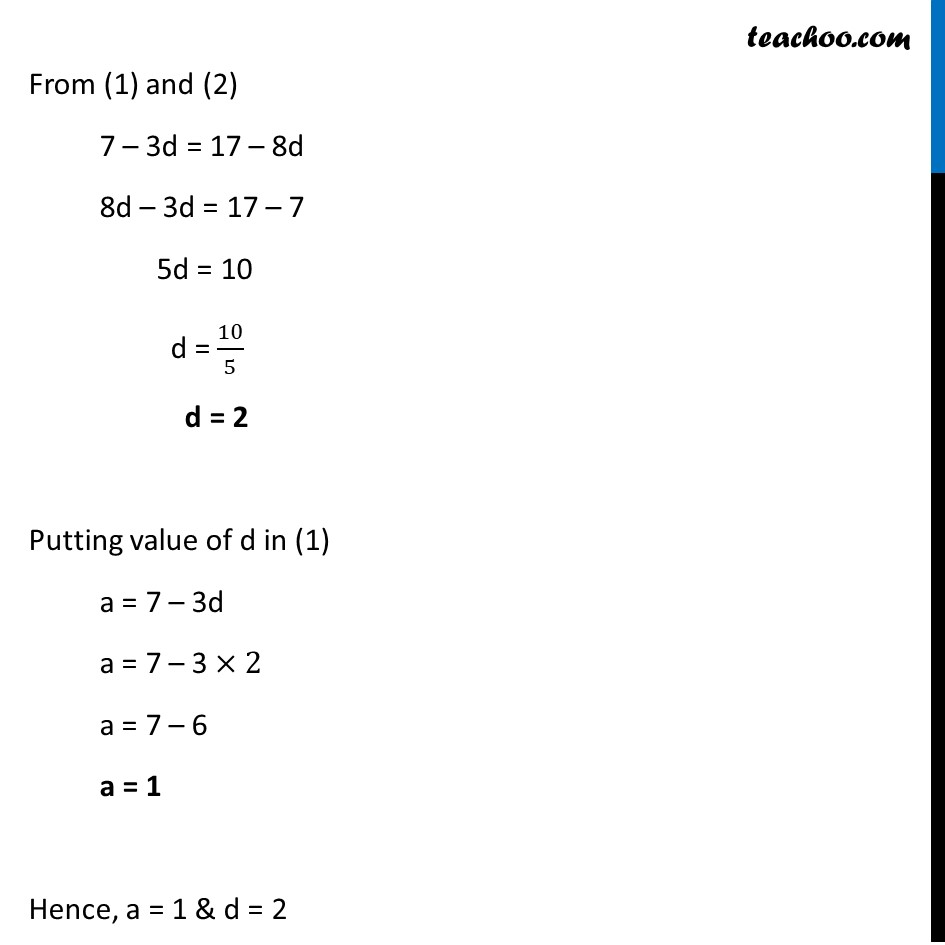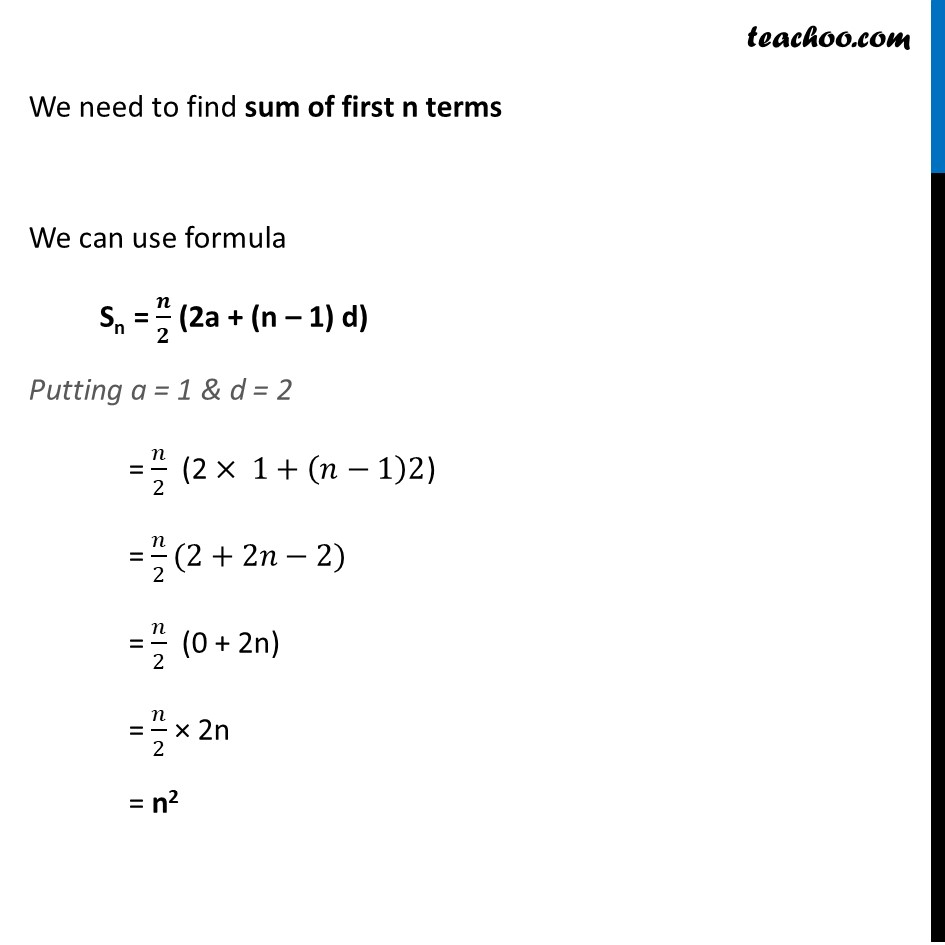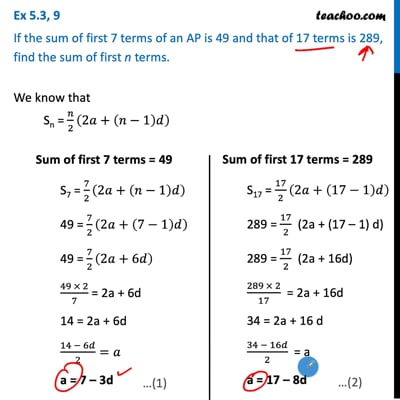Determining AP and finding sum

Chapter 5 Class 10 Arithmetic Progressions (Term 2)
Concept wiseThis video is only available for Teachoo black users

### Transcript

Ex 5.3, 9 If the sum of first 7 terms of an AP is 49 and that of 17 terms is 289, find the sum of first n terms. We know that Sn = 𝑛/2 (2𝑎+(𝑛−1)𝑑) Sum of first 7 terms = 49 S7 = 7/2 (2𝑎+(𝑛−1)𝑑) 49 = 7/2 (2𝑎+(7−1)𝑑) 49 = 7/2 (2𝑎+6𝑑) (49 × 2)/7 "= 2a + 6d" "14 = 2a + 6d" (14 − 6𝑑)/2=𝑎 a = 7 – 3d Sum of first 17 terms = 289 S17 = 17/2 (2𝑎+(17−1)𝑑) 289 = 17/2 (2a + (17 – 1) d) 289 = 17/2 (2a + 16d) (289 × 2)/17 = 2a + 16d 34 = 2a + 16 d (34 − 16𝑑)/2 = a a = 17 – 8d From (1) and (2) 7 – 3d = 17 – 8d 8d – 3d = 17 – 7 5d = 10 d = 10/5 d = 2 Putting value of d in (1) a = 7 – 3d a = 7 – 3 ×2 a = 7 – 6 a = 1 Hence, a = 1 & d = 2 We need to find sum of first n terms We can use formula Sn = 𝒏/𝟐 (2a + (n – 1) d) Putting a = 1 & d = 2 = 𝑛/2 (2 × 1+(𝑛−1)2) = 𝑛/2(2+2𝑛−2) = 𝑛/2 (0 + 2n) = 𝑛/2 × 2n = n2